# Metric LengthThis is Blublubox, a friendly alien from the planet BleBeep who is visiting the Earth. He'd like to find out about how Earthlings measure things like heights and distances. These are examples of length measurements.

Well, Blublubox, there are two main systems used for measuring things here on Earth. They are the metric system and the Imperial system (the US standard system is very similar to the Imperial system). In this article, we'll talk about measuring length using the metric system. This is the system that is now officially used in Australia and New Zealand. The childrens' grandparents might still use the Imperial system. You can read about the Imperial system in the article on measuring length in the Imperial system.

## Units for Length Measurement in the Metric System

There are many different units used for measuring length in the metric system. The most commonly used ones are:

• Millimetres
• Centimetres
• Metres
• Kilometres

### Millimetres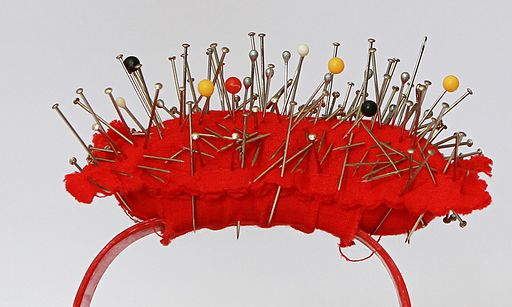Millimetres are the smallest of the commonly used metric units of length. They are used to measure very small lengths. They are the smallest of the commonly used metric length units. Carpenters often use millimetres when they are designing their furniture. This is because, for example, a very small difference in the lengths of the legs of a chair can cause it to wobble.

Some example measurements in millimetres are:

• A Blu-ray disc is $1$ millimetre thick.
• The head of a pin (the flat ones in the pin cushion) has a diameter of about $1$ millimetre.
• A five-cent coin is $1$ millimetre thick.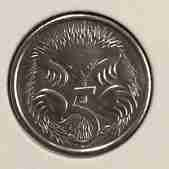• A flea (enlarged in the picture here) is somewhere between $1 \; \frac{1}{2}$ and $4$ millimetres long.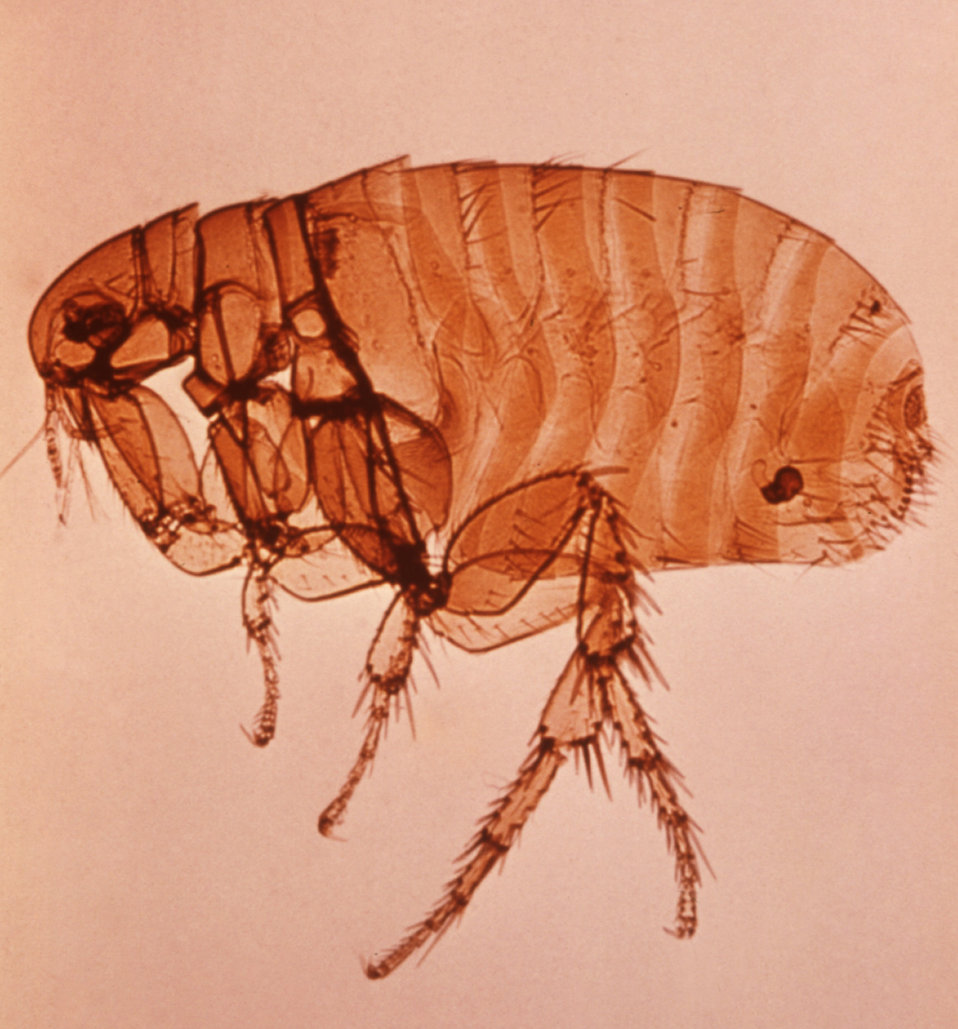### Centimetres

The centimetre is the next largest metric unit of length:

There are 10 millimetres in 1 centimetre.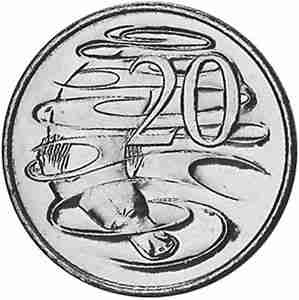Dressmakers often measure lengths in centimetres. We also measure our heights in centimetres.

Some examples of measurements in centimetres are

• A shirt button has a diameter of $1$ centimetre.
• A $5$ cent piece has a diameter of about $2$ centimetres, and a $20$ cent piece has a diameter of about $3$ centimetres.
• Your ruler is $30$ centimetres long.
• This pencil is $9$ centimetres long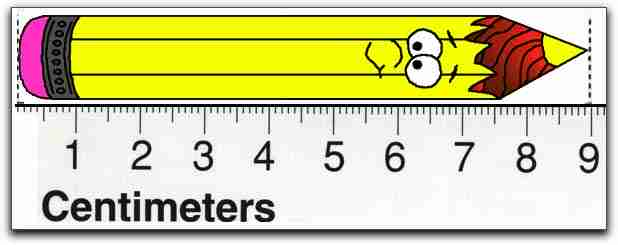• I am $165$ centimetres tall. Blublubox is about $93$ centimetres tall.

### Metres

The metre is the next largest metric unit of length:

There are 100 centimetres in 1 metre. There are also 1000 millimetres in 1 metre.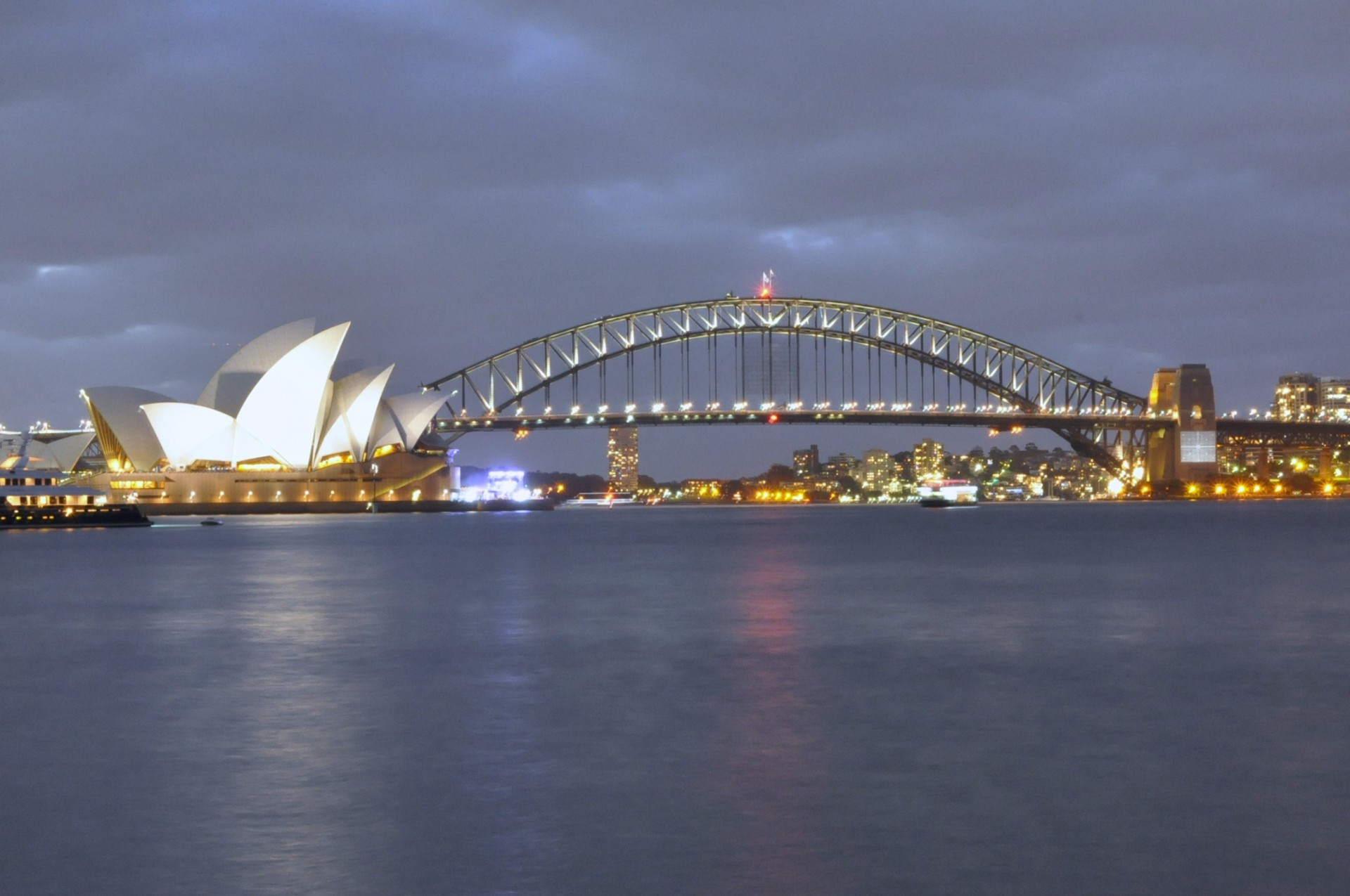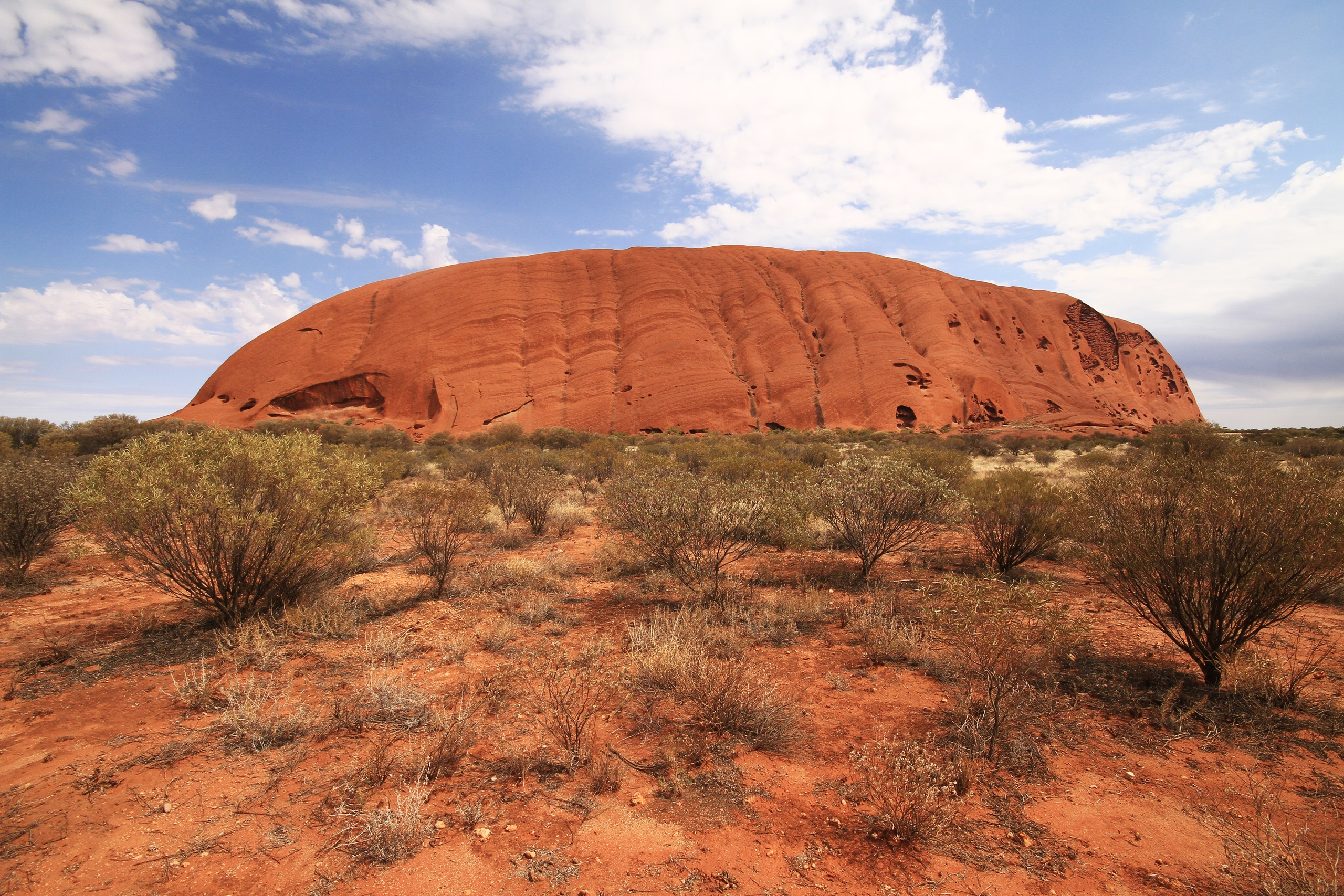We measure the heights of buildings and mountains in metres. We also use metres to measure the lengths of running tracks, playgrounds, rooms in houses and short paths.

Some examples of measurements in metres are

• A 4 or 5 year old child is about $1$ metre tall.
• The door knob on your door at home is $1$ metre above the floor.
• The shallow end of a swimming pool is around $1$ metre deep.
• A cricket pitch is about $20$ metres long and $3$ metres wide.
• The oval at the MCG is $171$ metres long.
• A female humpback whale is $15$-$16$ metres long.
• The Sydney Harbour Bridge is $134$ metres high.
• Uluru is $348$ metres high.

### Kilometres

The kilometre is the largest of the commonly used metric units of length. We use kilometres to measure long distances.

There are 1000 metres in 1 kilometre.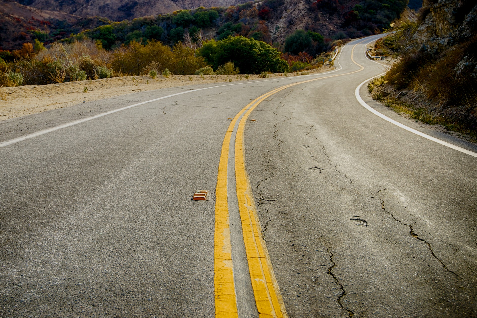We measure the distances between cities and the lengths of plane journeys in kilometres.

Some examples of measurements in kilometres are

• One kilometre is the distance you can walk in $12$-$15$ minutes.
• The maximum width of Bass Strait is $350$ kilometres.
• There are $878$ kilometres between Sydney and Melbourne along the M31.
• The maximum depth of the ocean is about $11$ kilometres.
• The Great Barrier Reef is $2,300$ kilometres long.
• The flight from Sydney to Wellington (NZ) is $2,224$ kilometres long.

### Conclusion

So, Blublubox, those are the commonly used metric measurements. You would measure the length of your eyelashes in millimetres, your height in centimetres, the height of your spaceship in metres, and the diameter of the Earth in kilometres.

### Description

This mini book covers the core of Math for Foundation, Grade 1 and Grade 2 mathematics including

1. Numbers
3. Subtraction
4. Division
5. Algebra
6. Geometry
7. Data
8. Estimation
9. Probability/Chance
10. Measurement
11. Time
12. Money
13. and much more

This material is provided free of cost for Parent looking for some tricks for their Prekinder, Kinder, Prep, Year 1 and Year 2 children

### Learning Objectives

These lessons are for kids aged 4-8 with the core objective to expose their brains to concepts of addition, subtraction, division, algebra and much more.

Author: Subject Coach
You must be logged in as Student to ask a Question.